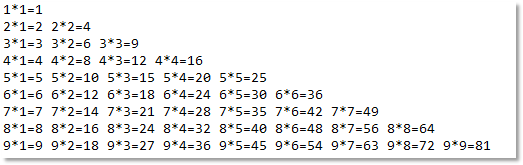# java循环语句之for

``````System.out.println("hello world!");
System.out.println("hello world!");
System.out.println("hello world!");
System.out.println("hello world!");
System.out.println("hello world!");
System.out.println("hello world!");
System.out.println("hello world!");
System.out.println("hello world!");
System.out.println("hello world!");
System.out.println("hello world!");
//...
//...``````

java中的循环语句共包括三个，分别是：for循环、while循环、do..while循环，接下来我们先来学习for循环。

for循环的语法格式如下图所示：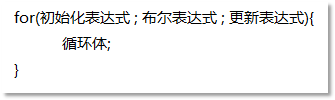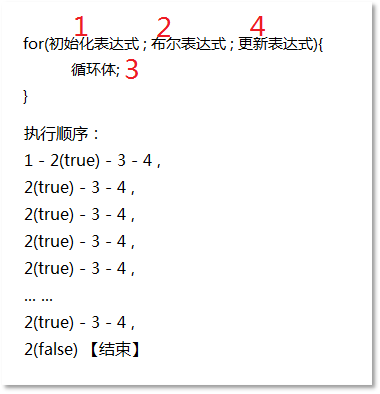``````for(;;){
System.out.print("死循环、");
}``````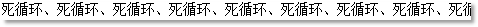``````for(int i = 1; i <= 10; i++){
System.out.println("i = " + i);
}``````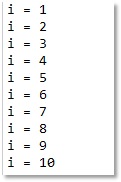``````//在for循环内部声明的变量i只在for循环中可见/有效
for(int i = 1; i <= 10; i++){
System.out.println("i = " + i);
}
//这行编译报错，i变量不存在
System.out.println("i = " + i);``````

``````public static void test(){
//变量的声明位置放到了for循环之外
int i = 1;
for(; i <= 10; i++){
System.out.println("i = " + i);
}
//这里就可以访问变量i了
System.out.println("i = " + i);
}``````

``````for(int i = 10; i > 0; i--){
System.out.println("i = " + i);
}
System.out.println("-------------分割线---------------");
for(int i = 10; i > 0; i -= 2){
System.out.println("i = " + i);
}
System.out.println("-------------分割线---------------");
for(int i = 100; i >= 10; i /= 3){
System.out.println("i = " + i);
}``````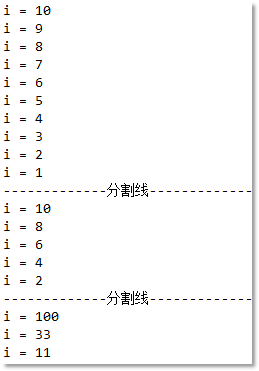``````int sum = 0;
for(int i = 1; i <= 100; i++){
sum += i;
}
System.out.println("sum = " + sum);``````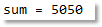``````int sum = 0;
for(int i = 1; i <= 100; i++){
if(i % 2 != 0){
sum += i;
}
}
System.out.println("sum = " + sum);``````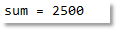``````int sum = 0;
for(int i = 1; i < 100; i += 2){
sum += i;
}
System.out.println("sum = " + sum);````````````//循环5次（B循环）
for(int i = 1;i <= 5; i++){
System.out.print("i = " + i + ",");
}

System.out.println();

//循环2次（A循环）
for(int j = 1;j <= 2; j++){
System.out.print("j = " + j + ",");
}

System.out.println();

//将B循环放到A循环体当中
for(int j = 1;j <= 2; j++){ //A循环(2次)

for(int i = 1;i <= 5; i++){ //B循环(5次)

System.out.print("i = " + i + ",");

}
}``````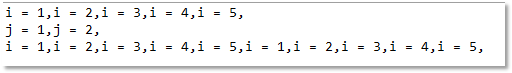图6-21：for循环嵌套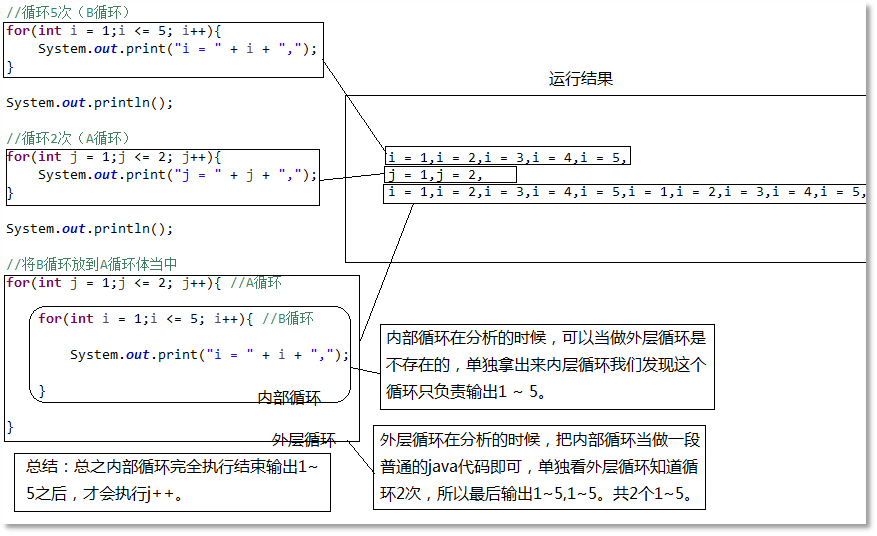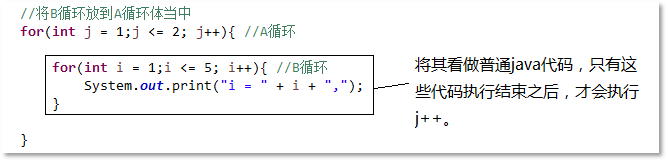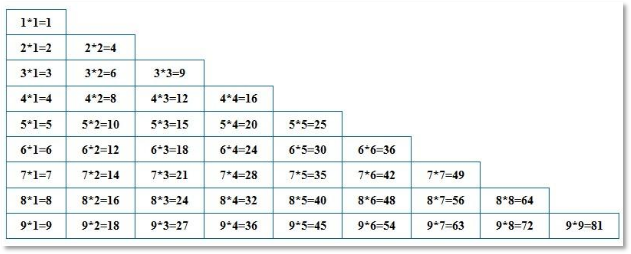``````public static void main(String[] args) {
for(int i = 1; i <= 9; i++){
System.out.println("i = " + i);
}
}``````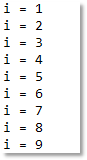``````for (int i = 1; i <= 9; i++) {

for (int j = 1; j <= i; j++) {

System.out.print(i * j + " ");

}

System.out.println();

}``````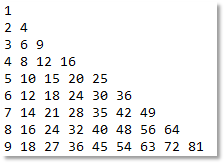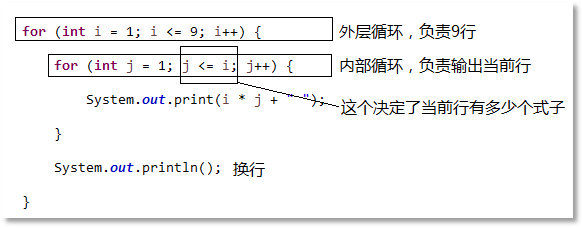``````for (int i = 1; i <= 9; i++) {

for (int j = 1; j <= i; j++) {

System.out.print(i + "*" + j + "=" + i * j + " ");

}

System.out.println();

}``````